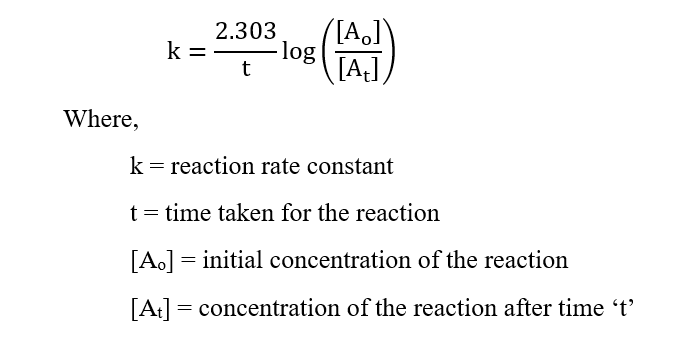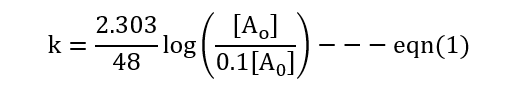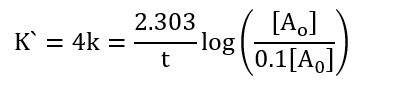# 57. In an experiment, a sample of NaClO3 was 90% decomposed in 48 min. Approximately how long would this decomposition have taken if the sample had been heated 20 °C higher? (Hint: Assume the rate doubles for each 10 °C rise in temperature.)

Question
5 views

57.

In an experiment, a sample of NaClO3 was 90% decomposed in 48 min. Approximately how long would this decomposition have taken if the sample had been heated 20 °C higher? (Hint: Assume the rate doubles for each 10 °C rise in temperature.)

check_circle

Step 1

Given:

Temperature is increased by 20 °C

Time to decompose = 48 min

Step 2

Assuming that the reaction is first order, the equation for first order reaction kinetics is:Step 3

Condition-1:Condition-2: When the temperature is increased by 20 oC, k increases by 4 times....

### Want to see the full answer?

See Solution

#### Want to see this answer and more?

Solutions are written by subject experts who are available 24/7. Questions are typically answered within 1 hour.*

See Solution
*Response times may vary by subject and question.
Tagged in